# JS算法：图文并茂讲解堆排序## 前言

### 理论

• Parent(i) = floor((i-1)/2)，i 的父节点下标
• Left(i) = 2i + 1，i 的左子节点下标
• Right(i) = 2(i + 1)，i 的右子节点下标

### 思路

• 此思路用于将任意数组调整成大根堆
• 首先将数组想象成是一个完美二叉树
• 我们的目的是将这个二叉数调整成大根堆，即每个节点的值都大于等于其左右孩子节点值
• 首先，寻找到最后一个根节点，此根节点有一个孩子是数组的最后一个元素
• 然后找出大的孩子节点与根节点对比，如果大的孩子节点比根节点大，则将根节点与大的孩子节点互换，保证根节点最大
• 接着向前遍历根节点
• 对每个根节点，都首先找出比较大的孩子节点，然后将大的孩子节点与根节点对比
• 如果孩子节点比根节点大，那么将孩子节点与根节点互换
• 然后将互换后得到新值的孩子节点作为新的根节点，将其与它自己的子节点再重复以上对比，以此进行深度遍历的重复操作，直到此相关树上的根节点都比孩子节点大为止
• 深度遍历操作完后，继续执行前面的根节点遍历操作，直到遍历到最后一个根节点

### 例子

• 现有数组 arr = [5,8,3,5,2,99,22,44]，将其调整为大根堆
• 数组可以表示堆即完美二叉树，因此将其转化成完美二叉树理解(此处可自行搜索，完美二叉树用数组表示)，如下图所示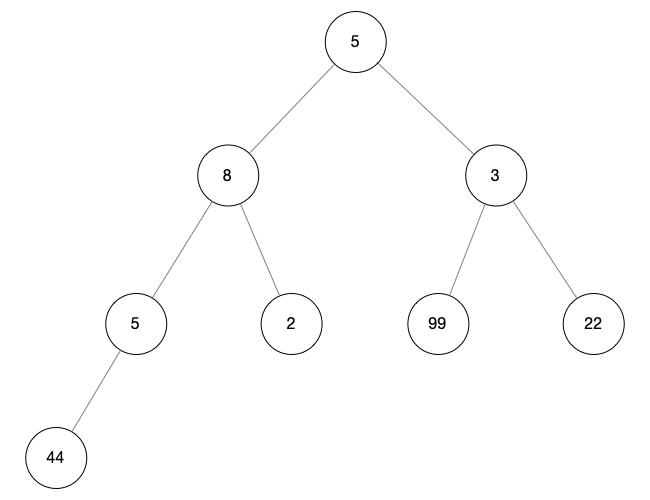0.png
• 寻找最后一个根节点，用 i 表示，从 arr.length / 2 作为初始值向前查找
• 当 i = arr.length / 2 = 4 时没有孩子节点，所以它不是根节点
• 向前遍历节点，当 i = 3 时，它拥有孩子节点 arr[arr.length - 1]，所以 arr = 5 就是我们想找的最后一个根节点
• 此时我们可以得最后一颗子树，如下图标记所示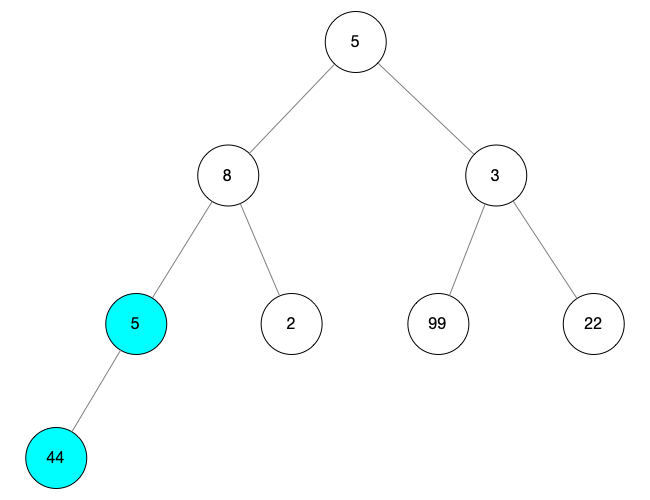图1.png
• 然后我们针对这颗子树进行调整操作

• 找到最大的孩子节点，用 child 表示，此时只有一个孩子节点 arr.length - 1，所以 child = arr.length - 1 = 7
• 将 child 与根节点（i = 3）对比，如果 child 的值 i 的值大，则互换值
• 此处 child 比 i 的值大所以互换值，child 的值改为 5，i 的值改为 44
• 由于 child 没有孩子节点所以不进行更深层次的遍历
• 操作完之后如下图所示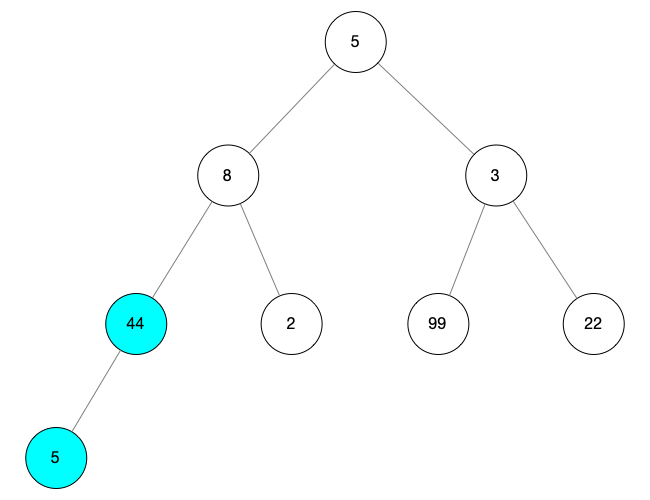2.png
• 然后向前继续寻找根节点，当 i = 2 的时候，我们将找到一颗新的子树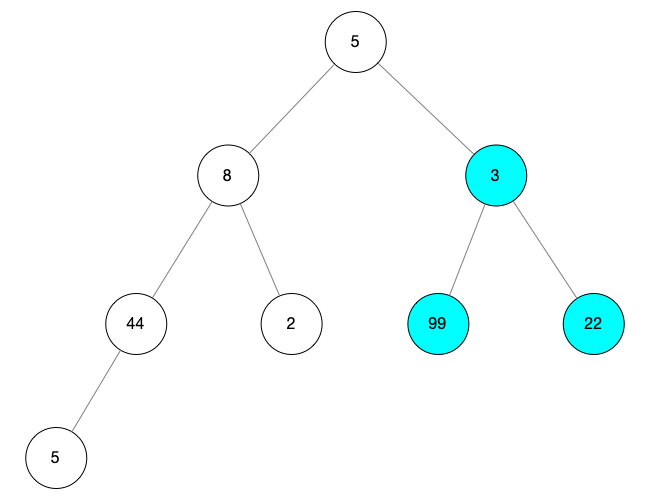3.png
• 对这颗找到的树进行调整操作

• 寻找大的孩子节点，用 child 表示，由图可知最大的孩子节点的值为 99，所以 child = 5
• 当前的根节点 i = 2，由于 child 的值比 i 的值大，所以互换
• 同时由于 child 后面没有孩子节点，所以结束操作
• 上面操作后可得到下图所示的树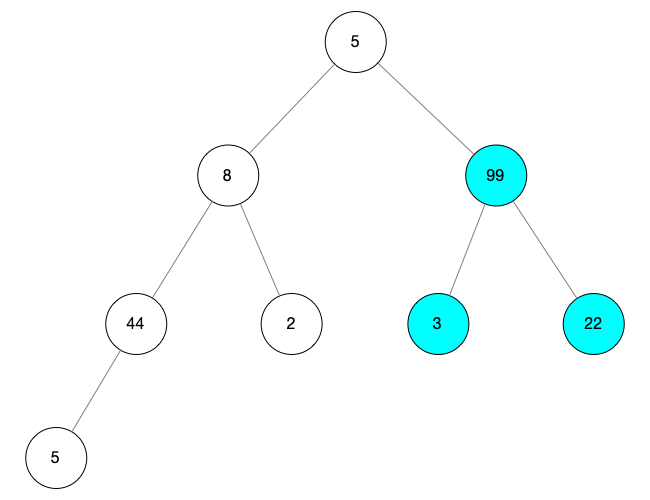4.png
• 继续向前寻找根节点，当 i = 1 的时候，我们找到一颗新的树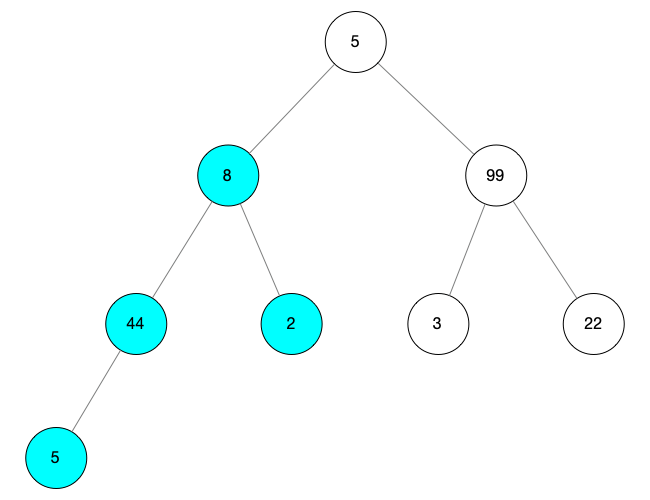5.png
• 对这颗树进行调整操作，此时 i = 1，child = 3

• 按照上面步骤，首先互换 child 和 i 的值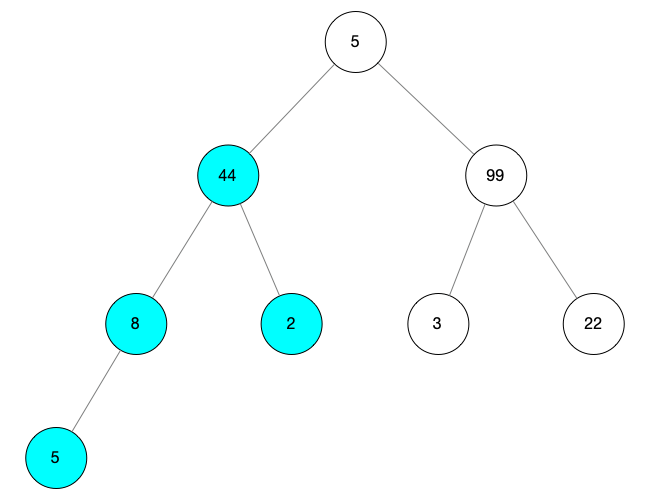6.png

• 然后由于 child 有孩子节点，所以将 i 指向 i，将 child 指向它自己的孩子节点
• 得到 i = 3， child = 7 重复比较操作，如果孩子节点比根节点大互换值
• 此处 arr[i] = 8，arr[child] = 5 根节点比孩子节点大，所以不替换
• 最终得到的树如下图所示6.png
• 继续向前遍历，得到最后一颗新树，根节点为 i = 0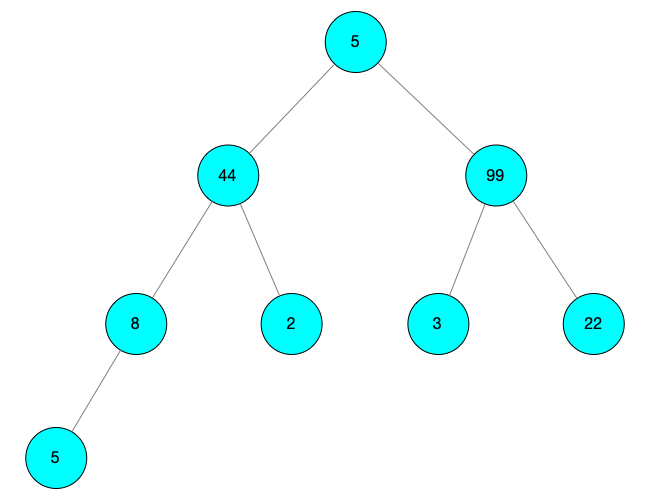7.png
• 对这颗树进行调整操作

• 此时 i = 0
• 先寻找 child = 2，arr[child] = 99
• arr[child] = 99 > arr[i] = 5，互换得到 arr[child] = 5，arr[i] = 99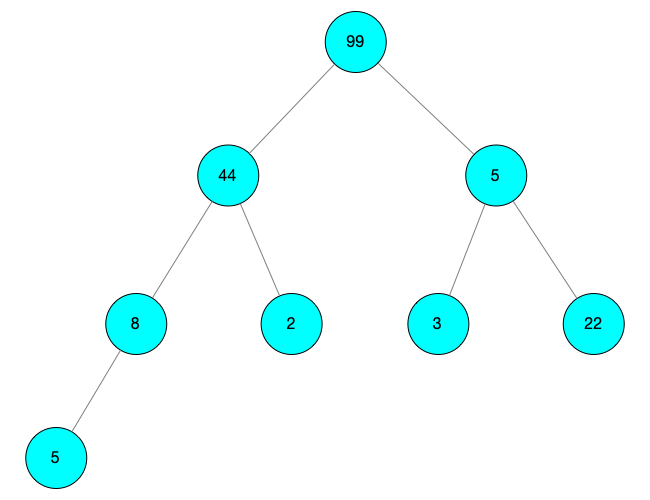8.png

• 由于 child 有孩子节点，所以对 child 的孩子节点进行深度调整
• i = child = 2，child = 2 * i + 1 = 5
• 由于此时有左右两个孩子节点，索引分别为 5 和 6，并且索引为 6 的节点值比较大，所以 child 更改为 6
• 比较 i 与 child 的值
• arr[i] = arr = 5 小于 arr[child] = arr = 22
• 所以进行值互换，得到 arr[i] = 22，arr[child] = 5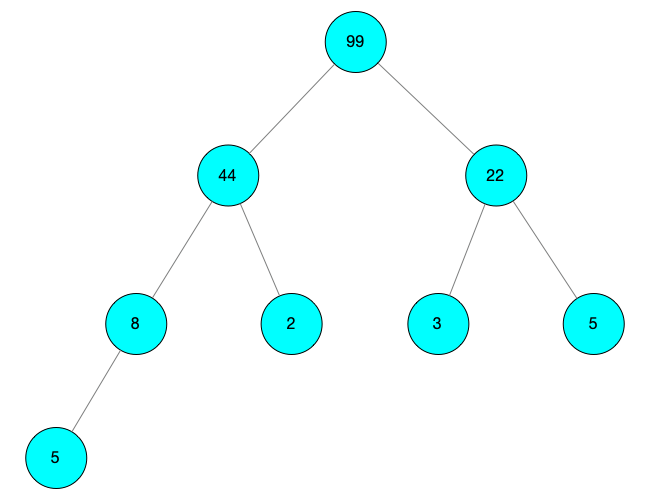9.png

• 此时 child 没有孩子节点，停止深度调整
• 最终得到大根堆如下图所示9.png
• 用数组进行表示则为：[99, 44, 22, 8, 2, 3, 5, 5]

### 代码

``/** *  此代码建议 mock 数据，并将其绘制成完美二叉树，参照二叉树进行阅读 */function buildHeap(data){    let len = data.length        // 从最后一个根节点开始，向前遍历所有根节点    // 取 len / 2 作为 i 的初始值，是因为最后一个孩子节点是 len - 1    // 它可能是左孩子也可能是右孩子，那么可以根据公式算出对应的根节点    // 它一定在 len / 2 附近，且小于 len / 2    for(let i = Math.floor(len / 2); i >= 0; i --){       heapAjust(data, i, len);    }}/** * 调整操作 * 根据传入的数据调整二叉树 * i 为根节点的 key * len 为需调整数据的长度 */function heapAjust(data, i, len){    // 寻找 i 的左孩子    let child = 2 * i + 1        // 如果 child 大于 len 说明 i 不是根节点    while(child < len) {                // 比较两个孩子节点，将 child 指向大的那个        if(child + 1 <= len && data[child] < data[child + 1]){            child = child + 1        }        // 如果孩子节点比根节点大，两个节点互换        if(data[i] < data[child]){                        let temp = data[i]            data[i] = data[child]            data[child] = temp             // 互换之后将被更新的孩子节点继续作为根节点，进行深度查找            i = child            child = 2 * i + 1        }        else {            break        }    }}``

## 堆排序

### 思路

• 先将整个数组调整成大根堆
• 则数组的第一个元素最大，将其与数组最后一个元素替换，此时大根堆被破坏
• 重新调整前 length - 1 个元素，将它们调整成新的大根堆
• 以此循环，直到堆中的元素只有一个时

### 代码

``var arraySort = function(arr) {    // 将数组调整成大根堆    buildHeap(arr);    // 下面需要注意的时候参数的边界    for(let i = arr.length - 1; i >= 0; i --) {        // 互换数据            let temp = arr[i]        arr[i] = arr        arr = temp                 // 将前面的元素重新调整成大根堆        heapAjust(arr, 0, i-1);    }}``

## 总结

• 堆排序是不稳定的
• 在寻找前几个值最大的场景中比较好用